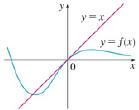Chapter 4.4, Problem 7E### Single Variable Calculus: Early Tr...

8th Edition
James Stewart
ISBN: 9781305270343

#### Solutions

Chapter
Section### Single Variable Calculus: Early Tr...

8th Edition
James Stewart
ISBN: 9781305270343
Textbook Problem

# The graph of a function f and its tangent line at 0 are shown. What is the value of lim x → 0   f ( x ) e x − 1 ?To determine

To evaluate: The value of limx0f(x)ex1 by using graph of f .

Explanation

Result used:

L’Hospital’s rule: For differentiable functions f and g where g0 and if the limit limxaf(x)g(x) is in indeterminate form then, limxaf(x)g(x)=limxaf(x)g(x) .

Given:

From the graph it can be identified that the equation of the tangent line of the graph f is y=x .

Calculation:

From the graph, line y=x is tangent to the curve f . So,  it represents first derivative of f.

Thus f(x)=1

### Still sussing out bartleby?

Check out a sample textbook solution.

See a sample solution

#### The Solution to Your Study Problems

Bartleby provides explanations to thousands of textbook problems written by our experts, many with advanced degrees!

Get Started

#### Domain Find the domain of the expression. 12. 1x1

Precalculus: Mathematics for Calculus (Standalone Book)

#### In problems 23-58, perform the indicated operations and simplify. 49.

Mathematical Applications for the Management, Life, and Social Sciences

#### Find for defined implicity by .

Study Guide for Stewart's Multivariable Calculus, 8th

#### The graph at the right has a local maximum at x ______. a) 1, 3, and 5 b) 2 c) 4 d) 2 and 4

Study Guide for Stewart's Single Variable Calculus: Early Transcendentals, 8th